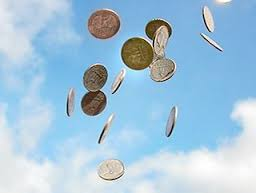Again the same old coins

Probability Level 1What is the expected number of heads in 101 tosses of a fair coin? If your answer comes in the form of $\dfrac{p}{q}$, find $p-q$.

×